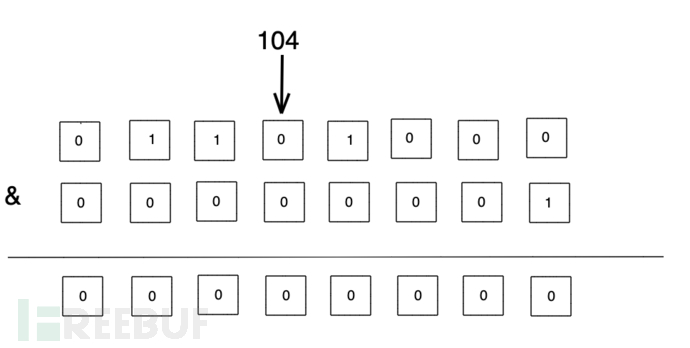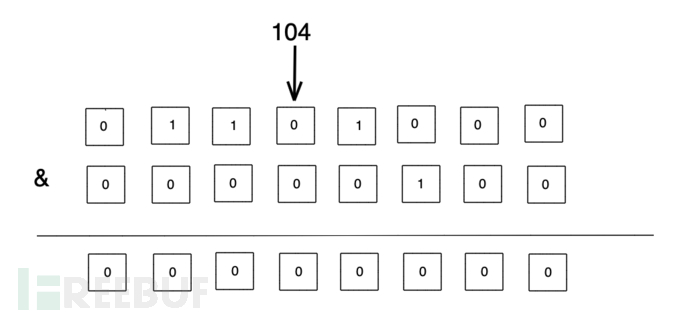SQL 注入是当前 Web 安全中最常见的安全问题之一，其危害性也比较大，众多白帽子在渗透测试过程中往往会首先着重进行 SQL 注入的测试。盲注是 SQL 注入的重要的技术之一，在现实中的 SQL 注入案例中，往往很难将注入的结果直接回显出来。因此，盲注也就成为了 SQL 注入必不可少的手段之一。本文想分享一个如何大大提升盲注效率的技巧。

1 & x = 0, x = 0

1 & x = 1, x = 1Talk is cheap, show me the code. 这里我们通过 python 的方式来实现：

def compute_by_and(word):
for ele in word:
ele_b, times = get_character(ele)
print(f"Guess the value {ele_b}:{chr(ele_b)} with {times} times")

def get_character(char):
char_b = ord(char)
value = 0
times = 0
for i in range(7):
times = times + 1
if char_b & (2 ** i):
value = value + (2 ** i)
return value, times

if name == "main":
compute_by_and("hello")

import string

def brute_force(word):
times = 0
for ele in word:
for c in string.printable:
times = times + 1
if ele == c:
break
print(f"Brute force {word} with {times} times")

if name == "main":
brute_force("hello_world")
myapp myapp_card myapp_card_perform